# 1. Intro to graphs

Graph: vertices connected by edges.

terminology:

• path: sequence of vertices connected by edges
• cycle: path with same starting and ending vertex
• two vertices are connected: if there is a path between

ex of graph problems:

• path: or connectivity
• shortest path
• cycle
• Euler tour (ouii..)
• Hamilton tour
• MST
• bi-connectivity: is there a vertex whose removal disconnects the graph?
• planarity
• isomorphism

# 2. Graph API

## graph representation

• vertex representation: use integers between 0 and V-1
• anormalies: self-loop and multiple edges are possible
```public class Graph{
Graph(int V);
int V();// nb of vertices
int E();// nb of edges
}
```

print all edges: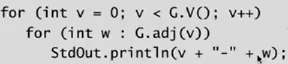basic functions:

```static int degree(Graph g, int v){
int deg = 0;
return deg;
}
static int nbOfSelfloops(Graph g){
int cnt = 0;
for(int v=0; v<G.V(); v++)
if (w==v) cnt++;
return cnt/2;
}
```

## edge representation

• set-of-edge implementation: a list of all edges ⇒ can lead to inefficient implementation
• adj-matrix implementation: maintain a 2d (V*V) boolean array ⇒ space complexity too heavy
• adj-list implementation: vertex-indexed array, each array entry is a `Bag` (类似桶bucket) ⇒ sutable for sparse graphs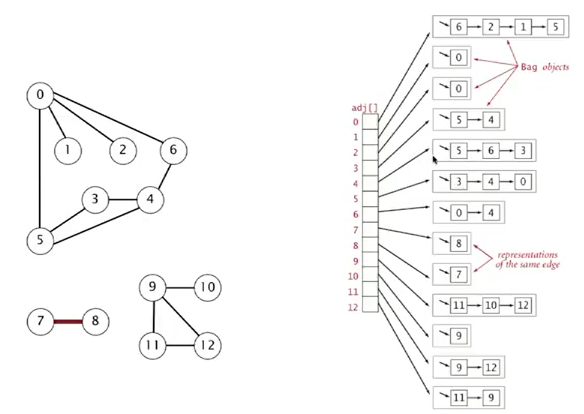```private final int V;
public Graph(int V){
this.V = V;
this.adj = (Bag<Integer>[]) new Bag[V];// java cannot create generic array
for(int v = 0; v<V; v++) adj[v] = new Bag<Integer>();
}
}
```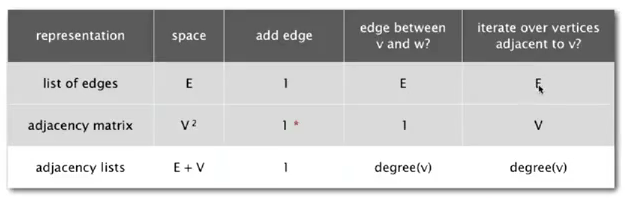# 3. Depth-First Search

Tremaux maze exploration: trace back when no unvisited vertices availiable.

DFS goal: systematically search through a graph.
design pattern: decouple graph data and graph processing.

```public class Paths{
Path(Graph G, int s);// graph G and source s
boolean hasPathTo(int v);
Iterable<Integer> pathTo(int v);
}
```

algo: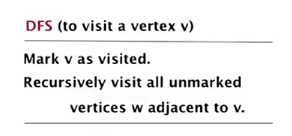## implementation

• 用一个boolean数组`visited[]`作为标记
• 为了找到一条具体的路径(ie, 一系列节点), 维护一个`prev[]`数组, 存放当前节点是从哪个节点走过来的.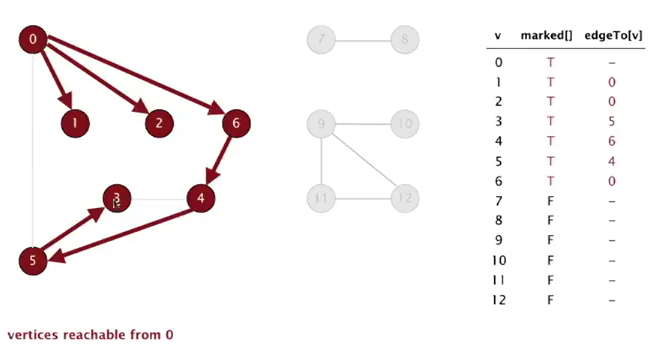```//public class DFSpaths extends Paths...
boolean[] visited = new boolean[V];
int[] prev = new int[V];
public void dfs(int v){
visited[v] = true;
if(!visited[w]) {
prev[w]=v;
dfs(w);
}
}
public Iterable<Integer> pathTo(int v){
Stack<Integer> s = new Stack<Integer>();
for(int x = v; x!=s; x = prev[x])
s.push(x);
return s;
}
```

## properties

prop.
DFS visite all edges in time propotional to the sum of their degrees(ie. nb of edges).

not recursive algo.
maintain a queue, add to queue for all vertices not-marked.

## implementation

• use `visited[]` to mark vertices
• use a `prev[]` array to get explicit path
• use a `dist[]` array to record the shortest dist from v to source (can use `dist` to replace `visited`)
```public void bfs(Graph G, int s){
boolean visited[] = new boolean[G.V()];
int prev[] = new int[G.V()];
int dist[] = new int[G.V()];
Queue<Integer> q = new Queue<Integer>();
visited[s] = true;
q.push(s);
while(!q.isEmpty()){
int v = q.dequeue();
if(!visited[w]) {
prev[w] = v;
visited[w] = true;
q.enqueue(w);
}
}
}
```

## property

prop.
BFS computes the shortest path from s to all vertices using time propotional to E+V.

intuition: BFS examines nodes by increasing distance

# 5. Connected Components

dealing with connectivity(equivalence) queries ⇒ answer in constant time (with preprocessing).

```public class CC{
boolean connected(int v, int w);
int count();// nb of CCs
int id(int v);//id for a CC
}
```

⇒ Union-Find ? ⇒ Use DFS!!

def. connected component is a maximal set of connected vertices.

algo: for each unmarked vertex, run dfs(with increasing cc id)...
after the preprocessing, we can get the array `id[]` and cc count `cnt`...

# 6. Graph Challenges

some typical pbs
pb1. bipartite graph

Can we divide vertices into 2 subsets, where all edge go from one subset to other.
⇒ can be done with dfs. cf. booksite

pb2. cycle detection
⇒ simple using dfs.

pb3. Euler cycle
Find a cycle that uses all edges exactely once.
[Euler] a graph is Eulerian iff all vertices have even degree.
⇒ typical diligent algo students can do. cf. booksite

pb4. Hamilton cycle
Find cycle that visits each vertex exactly once.
⇒ intractable (typical NP-complete pb)

pb5. isomorphism of graphs
Are two graphs identical except for vertex names?
⇒ no one knows...

pb6. planary graph
Lay out a graph in the plane without crossing edges?
⇒ expert level. exists linear time algo based on DFS by Tarjan, but too complicated.